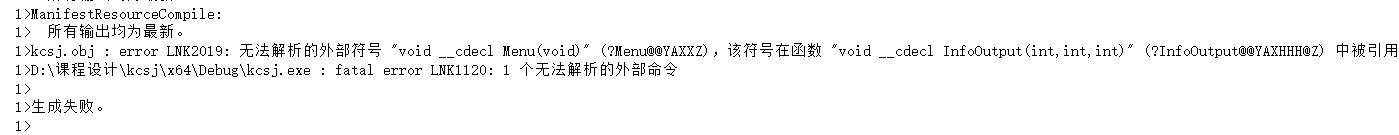1>kcsj.obj : error LNK2019: 无法解析的外部符号 "void __cdecl Menu(void)" (?Menu@@YAXXZ)，该符号在函数 "void __cdecl InfoOutput(int,int,int)" (?InfoOutput@@YAXHHH@Z) 中被引用
1>D:\课程设计\kcsj\x64\Debug\kcsj.exe : fatal error LNK1120: 1 个无法解析的外部命令# include < iostream >

using namespace std;

# define NULL 0

typedef struct
{
char proname; //项目名称
int pronum; //项目编号
int top; //取前3名或前5名积分，由用户自己定义
int range; //名次
int mark; //分数
}Pronode;

typedef struct
{
char schname; //学校名称
int schnum; //学校编号
int score; //总分
int Mscore; //男团体总分
int Wscore; //女团体总分

Pronode a[M+W]; //项目数组
}Schnode;

Schnode s[N];

{
cout<<" *************************************************"<<endl;
cout<<" ***** 欢迎使用 *****"<<endl;
cout<<" ***** 运动会分数统计系统 *****"<<endl;
cout<<" *************************************************"<<endl;
cout<<endl;
}

{
cout<<" *************************************************"<<endl;
cout<<" ***** 主菜单 *****"<<endl;
cout<<" *************************************************"<<endl;
cout<<" ***** 1.输入信息 *****"<<endl;
cout<<" ***** 2.输出信息 *****"<<endl;
cout<<" ***** 3.查询信息 *****"<<endl;
cout<<" ***** 4.调用信息 *****"<<endl;
cout<<" ***** 5.关 于 *****"<<endl;
cout<<" ***** 6.退出系统 *****"<<endl;
cout<<" *************************************************"<<endl;
cout<<" ***** 注：第一次运行本程序时请选择1号功能 *****"<<endl;
cout<<" *************************************************"<<endl;
}

{
cout<<" *************************************************"<<endl;
cout<<" ***** 运动会总分显示菜单 *****"<<endl;
cout<<" *************************************************"<<endl;
cout<<" ***** 1.按学校名称输出 *****"<<endl;
cout<<" ***** 2.按学校总分输出 *****"<<endl;
cout<<" ***** 3.按男团总分输出 *****"<<endl;
cout<<" ***** 4.按女团总分输出 *****"<<endl;
cout<<" ***** 5.退出返回主菜单 *****"<<endl;
cout<<" ***** 6.直接退出本系统 *****"<<endl;
cout<<" *************************************************"<<endl;
}

void InitSch() //初始化信息
{
int i;
for(i=0;i<N;i++)
{
s[i].score=0;
s[i].Mscore=0;
s[i].Wscore=0;
}
}

void InfoInput(int n,int m,int w) //信息输入模块
{
int i,j,k,p;
InitSch(); //初始化信息
for(i=0;i {
cout cin>>s[i].schname;
cout<<" 学校编号：";
cin>>s[i].schnum;
for(j=0;j {
cout cin>>s[i].a[j].proname;
cout<<" 项目编号：";
cin>>s[i].a[j].pronum;
cout<<" 取前3名还是前5名：";
cin>>s[i].a[j].top;
cout<<" 此项目获得的名次个数：";
cin>>k;
for(p=0;p {
s[i].a[j].range[p]=0;
s[i].a[j].mark[p]=0;
}
for(p=0;p {
cout cin>>s[i].a[j].range[p];
if(s[i].a[j].top==3) //匹配各名次对应的分数
{
switch(s[i].a[j].range[p])
{
case 0: s[i].a[j].mark[p]=0; break;
case 1: s[i].a[j].mark[p]=5; break;
case 2: s[i].a[j].mark[p]=3; break;
case 3: s[i].a[j].mark[p]=2; break;
}
}
else
{
switch(s[i].a[j].range[p])
{
case 0: s[i].a[j].mark[p]=0; break;
case 1: s[i].a[j].mark[p]=7; break;
case 2: s[i].a[j].mark[p]=5; break;
case 3: s[i].a[j].mark[p]=3; break;
case 4: s[i].a[j].mark[p]=2; break;
case 5: s[i].a[j].mark[p]=1; break;
}
}
s[i].score=s[i].score+s[i].a[j].mark[p]; //统计学校总分
if(j<=m-1)
s[i].Mscore=s[i].Mscore+s[i].a[j].mark[p]; //统计男团总分
else
s[i].Wscore=s[i].Wscore+s[i].a[j].mark[p]; //统计女团总分
}
cout<<endl;
}
}

}

void Output(int b[],int n) //输出各学校运动会分数信息
{
int i;
for(i=0;i<n;i++)
{
cout<<" 学校名称："<<s[b[i]].schname<<endl;
cout<<" 学校编号："<<s[b[i]].schnum<<endl;
cout<<" 学校总分："<<s[b[i]].score<<endl;
cout<<" 男团总分："<<s[b[i]].Mscore<<endl;
cout<<" 女团总分："<<s[b[i]].Wscore<<endl;
cout<<endl;
}

system("pause"); //程序暂停，以显示结果
system("cls"); //清屏
}

void InfoOutput(int n,int m,int w) //信息输出模块
{
FILE *fp;
int number,i,j,k,b[N];
if((fp=fopen("sport.txt","r"))==NULL) //以读方式打开文本文件
//并判定能否正常打开
{
cout<<" 文件打不开!"< exit(0); //调用函数exit终止程序运行
}
while(1)
{
cout cin>>number;
switch(number)
{
case 1: //按学校名称输出
system("cls");
cout<<" 运动会各学校信息按学校名称输出如下："<<endl;
for(i=0;i<n;i++) //输出各学校运动会分数信息
{
cout<<" 学校名称："<<s[i].schname<<endl;
cout<<" 学校编号："<<s[i].schnum<<endl;
cout<<" 学校总分："<<s[i].score<<endl;
cout<<" 男团总分："<<s[i].Mscore<<endl;
cout<<" 女团总分："<<s[i].Wscore<<endl;
cout<<endl;
}
system("pause"); //程序暂停，以显示结果
system("cls"); //清屏
break;
case 2: //按学校总分输出
system("cls");

cout<<" 运动会各学校信息按学校总分输出如下："<<endl;
for(i=0;i<n;i++) //冒泡排序，用辅助数组记住学校结点的下标
b[i]=i;
for(i=0;i<n;i++)
{
for(j=i+1;j<n;j++)
{
if(s[b[i]].score < s[j].score)
{
k=b[i];
b[i]=b[j];
b[j]=k;
}
}
}
Output(b,n); //按所记下标的顺序输出各学校运动会分数信息

break;
case 3: //按男团总分输出
system("cls");

cout<<" 运动会各学校信息按男团总分输出如下："<<endl;
for(i=0;i<n;i++) //冒泡排序，用辅助数组记住学校结点的下标
b[i]=i;
for(i=0;i<n;i++)
{
for(j=i+1;j<n;j++)
{
if(s[b[i]].Mscore < s[j].Mscore)
{
k=b[i];
b[i]=b[j];
b[j]=k;
}
}
}
Output(b,n); //按所记下标的顺序输出各学校运动会分数信息
break;
case 4: //按女团总分输出
system("cls");

cout<<" 运动会各学校信息按女团总分输出如下："<<endl;
for(i=0;i<n;i++) //冒泡排序，用辅助数组记住学校结点的下标
b[i]=i;
for(i=0;i<n;i++)
{
for(j=i+1;j<n;j++) //n m w ????
{
if(s[b[i]].Wscore < s[j].Wscore)
{
k=b[i];
b[i]=b[j];
b[j]=k;
}
}
}
Output(b,n); //按所记下标的顺序输出各学校运动会分数信息
break;
case 5: //退出返回主菜单
system("cls");

break;
case 6: //退出系统
exit(0);
default:
cout<<" 对不起，无此功能，请输入正确的功能序号!"<<endl;
system("pause");
system("cls");
InfoOutput(n,m,w);
break;

}
}
fclose(fp); //关闭文件
}

{
cout<<" *************************************************"<<endl;
cout<<" ***** 1.按学校编号查询 *******"<<endl;
cout<<" ***** 2.按项目编号查询 *******"<<endl;
cout<<" ***** 3.退出返回主菜单 *******"<<endl;
cout<<" ***** 4.直接退出本系统 *******"<<endl;
cout<<" *************************************************"<<endl;

}

void Inquiry(int n,int m,int w) //信息查询模块
{
int number,i,j,k,y;
while(1)
{

cout<<" 请选择查询方式：";
cin>>number;
switch(number)
{
case 1: //按学校编号查询
system("cls");

cout<<" 要查询的学校编号：";
cin>>i;
if(i>n) //学校编号超出范围，输出提示信息
cout<<" 这个学校没有参加此次运动会!"< else
{
cout cin>>j;
if(j>m+w || j==0) //项目编号超出范围，输出提示信息
cout<<" 此次运动会没有这个项目!"< else //输出要查询学校项目的成绩
{
cout for(k=0;k {
if(s[i-1].a[j-1].range[k]!=0)
cout if(s[i-1].a[j-1].mark[k]!=0)
cout }
}
}
system("pause");
system("cls");
break;
case 2: //按项目编号查询
system("cls");
cout cin>>y;
if(y>m+w || y==0) //项目编号超出范围，输出提示信息
cout<<" 此次运动会没有这个项目!"<<endl;
else //输出该项目取得名次的学校的成绩
{
cout<<" 该项目取前"<<s.a[y-1].top<<"名，取得该名次的学校："<<endl;
for(i=0;j<n;i++)
for(j=0;j<5;j++)
{
if(s[i].a[y-1].range[j]!=0)
cout<<" 学校名称："<<s[i].schname<<" 学校编号："<<s[i].schnum<<" 名次："<<s[i].a[y-1].range[j];
if(s[i].a[y-1].mark[j]!=0)
cout<<" 分数："<<s[i].a[y-1].mark[j]<<endl;
}
}
system("pause");

system("cls");

break;
case 3: //退出返回主菜单
system("cls");

break;
case 4: //退出系统
exit(0);
default:
cout<<" 对不起，无此功能，请输入正确的功能序号!"<<endl;
system("pause");
system("cls");
Inquiry(n,m,w);
break;
}
}
}

{
cout<<" 啦啦啦"<<endl;
}

void fsave() //保存信息，即将信息写入文件里
{
FILE *fp;
if((fp=fopen("sport.txt","w"))==NULL) //以写方式打开文本文件
//并判定能否正常打开
{
cout<<" 文件打不开!"<<endl; //不能正常打开文件的处理
exit(0); //调用函数exit终止程序运行
}
fwrite(s, sizeof(Schnode), N, fp); //调用文件数据块写函数将信息写进文件里
fclose (fp); //关闭文件
}

{
FILE *fp;
int i,j,m;
if((fp=fopen("sport.txt","r"))==NULL) //以读方式打开文本文件
//并判定能否正常打开
{
cout<<" 文件打不开!"<<endl; //不能正常打开文件的处理
exit(0); //调用函数exit终止程序运行
}
for(i=0;i<N;i++) //将读出的信息输出
{
cout<<" 学校名称："<<s[i].schname<<endl;
cout<<" 学校编号："<<s[i].schnum<<endl;
for(j=0;j<M+W;j++)
{
cout<<" 项目名称："<<s[i].a[j].proname<<endl;
cout<<" 项目编号："<<s[i].a[j].pronum<<endl;
cout<<" 取前3名还是前5名："<<s[i].a[j].top<<endl;
for(m=0;m<5;m++)
{
if(s[i].a[j].range[m]!=0)
cout<<" 名次："<<s[i].a[j].range[m];
if(s[i].a[j].mark[m]!=0)
cout<<" 分数："<<s[i].a[j].mark[m]<<endl;
}
cout<<endl;
}
}
fclose(fp); //关闭文件
}

void Menu(int n,int m,int w) //主菜单函数

{
int number;
do
{
cout<<" 请选择：";
cin>>number;
switch(number)
{
case 1: //输入信息
system("cls");

cout<<" 请输入运动会各学校信息："<<endl;
InfoInput(n,m,w); //信息输入模块
fsave(); //保存信息
system("cls");

break;
case 2: //输出信息
system("cls");

InfoOutput(n,m,w); //信息输出模块
break;
case 3: //查询信息
system("cls");

Inquiry(n,m,w); //信息查询模块
break;
case 4: //调用信息
system("cls");

system("pause");

system("cls");

break;
case 5: //“关于”模块
system("cls");

system("pause");

system("cls");
break;
case 6: //退出系统
system("cls");
cout<<" 谢谢使用!"<<endl;
exit(0);
default: //其他
cout<<" 对不起，无此功能，请输入正确的功能序号!"<<endl;
system("pause");

system("cls");
break;
}
}while(1);

}

void main() //主函数
{

int n,m,w;
cout<<"学校个数：";
cin>>n;
cout<<"男子项目个数：";
cin>>m;
cout<<"女子项目个数：";
cin>>w;
}

2个回答sleeeep 回复贵阳挖掘机马善福，自备车辆专业挖游泳池: 非常感谢sleeeep 你好 我附上了代码 能麻烦你帮我看看吗 非常感谢